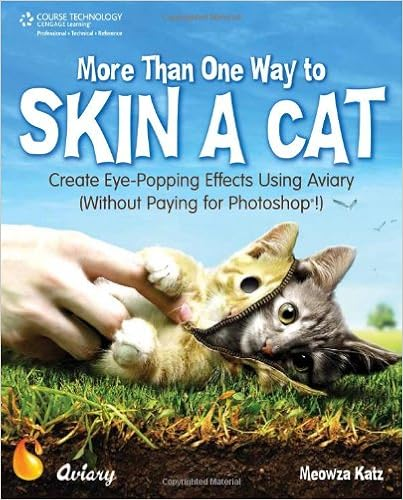# My Favorite One-Liners: Part 79

In this series, I’m compiling some of the quips and one-liners that I’ll use with my students to hopefully make my lessons more memorable for them.

I’ll use today’s quip when there are multiple reasonable ways of solving a problem. For example,

Two fair dice are rolled. Find the probability that at least one of the rolls is a six.

This can be done by directly listing all of the possibilities:$11 \qquad 12 \qquad 13 \qquad 14 \qquad 15 \qquad 16$$21 \qquad 22 \qquad 23 \qquad 24 \qquad 25 \qquad 26$$31 \qquad 32 \qquad 33 \qquad 34 \qquad 35 \qquad 36$$41 \qquad 42 \qquad 43 \qquad 44 \qquad 45 \qquad 46$$51 \qquad 52 \qquad 53 \qquad 54 \qquad 55 \qquad 56$$61 \qquad 62 \qquad 63 \qquad 64 \qquad 65 \qquad 66$

Of these 36 possibilities, 11 have at least one six, so the answer is$11/36$.

Alternatively, we could use the addition rule:$P(\hbox{first a six or second a six}) = P(\hbox{first a six}) + P(\hbox{second a six}) - P(\hbox{first a six and second a six})$$= P(\hbox{first a six}) + P(\hbox{second a six}) - P(\hbox{first a six}) P(\hbox{second a six})$$= \displaystyle \frac{1}{6} + \frac{1}{6} - \frac{1}{6} \times \frac{1}{6}$$= \displaystyle \frac{11}{36}$.

Another possibility is using the complement:$P(\hbox{at least one six}) = 1 - P(\hbox{no sixes})$$= 1 - P(\hbox{first is not a six})P(\hbox{second is not a six})$$= 1 - \displaystyle \frac{5}{6} \times \frac{5}{6}$$= \displaystyle \frac{11}{36}$

To emphasize that there are multiple ways of solving the problem, I’ll use this one-liner:

There are plenty of ways to skin a cat… for those of you who like skinning cats.

When I was a boy, I remember seeing some juvenile book of jokes titled “1001 Ways To Skin a Cat.” A recent search for this book on Amazon came up empty, but I did find this:## 2 thoughts on “My Favorite One-Liners: Part 79”

This site uses Akismet to reduce spam. Learn how your comment data is processed.